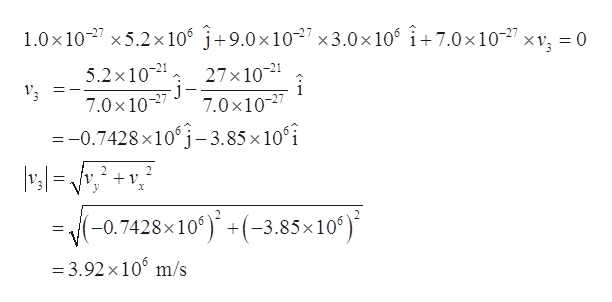# An unstable nucleus of mass 1.7 ✕ 10−26 kg, initially at rest at the origin of a coordinate system, disintegrates into three particles. One particle, having a mass of m1 = 1.0 ✕ 10−27 kg,moves in the positive y-direction with speed v1 = 5.2 ✕ 106 m/s.Another particle, of mass m2 = 9.0 ✕ 10−27 kg,moves in the positive x-direction with speed v2 = 3.0 ✕ 106 m/s.Find the magnitude and direction of the velocity of the third particle. (Assume that the +x-axis is to the right and the +y-axis is up along the page.)

Question
151 views

An unstable nucleus of mass 1.7 ✕ 10−26 kg, initially at rest at the origin of a coordinate system, disintegrates into three particles. One particle, having a mass of
m1 = 1.0 ✕ 10−27 kg,
moves in the positive y-direction with speed
v1 = 5.2 ✕ 106 m/s.
Another particle, of mass
m2 = 9.0 ✕ 10−27 kg,
moves in the positive x-direction with speed
v2 = 3.0 ✕ 106 m/s.
Find the magnitude and direction of the velocity of the third particle. (Assume that the +x-axis is to the right and the +y-axis is up along the page.)

check_circle

Step 1

Given values:

Mass of the nucleus, M = 1.7 ✕ 10-26 kg = 17.0 ✕ 10-27 kg

m1 = 1.0 ✕ 10-27 kg,
v1 = 5.2 ✕ 106 m/s.          (In positive Y - direction)
m2 = 9.0 ✕ 10-27 kg,

v2 = 3.0 ✕ 106 m/s.           (In positive X - direction)

Step 2

Let the velocity of the third particle be v3

Mass of the third particle m3 = mass of the nucleus – mass of the other two particle

= 17.0 ✕ 10-27 - (1.0 ✕ 10-27 + 9.0 ✕ 10-27)

m3 = 7✕ 10-27 kg

Step 3

Total initial momentum = 0                                                            (Nucleus is at rest)

Total final momentum = P1...help_outlineImage Transcriptionclose1.0x 10 5.2x 106 j+9.0x1027 x3.0x 106 i-+ 7.0 x 10-27 5.2x102 27x102 j 7.0x 1027 7.0x10-27 =-0.7428 x10 j-3.85x10 i X +v (-0.7428x10)(-3.85 x 10) =3.92 x 106 m/s fullscreen

### Want to see the full answer?

See Solution

#### Want to see this answer and more?

Solutions are written by subject experts who are available 24/7. Questions are typically answered within 1 hour.*

See Solution
*Response times may vary by subject and question.
Tagged in

### Physics